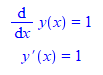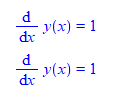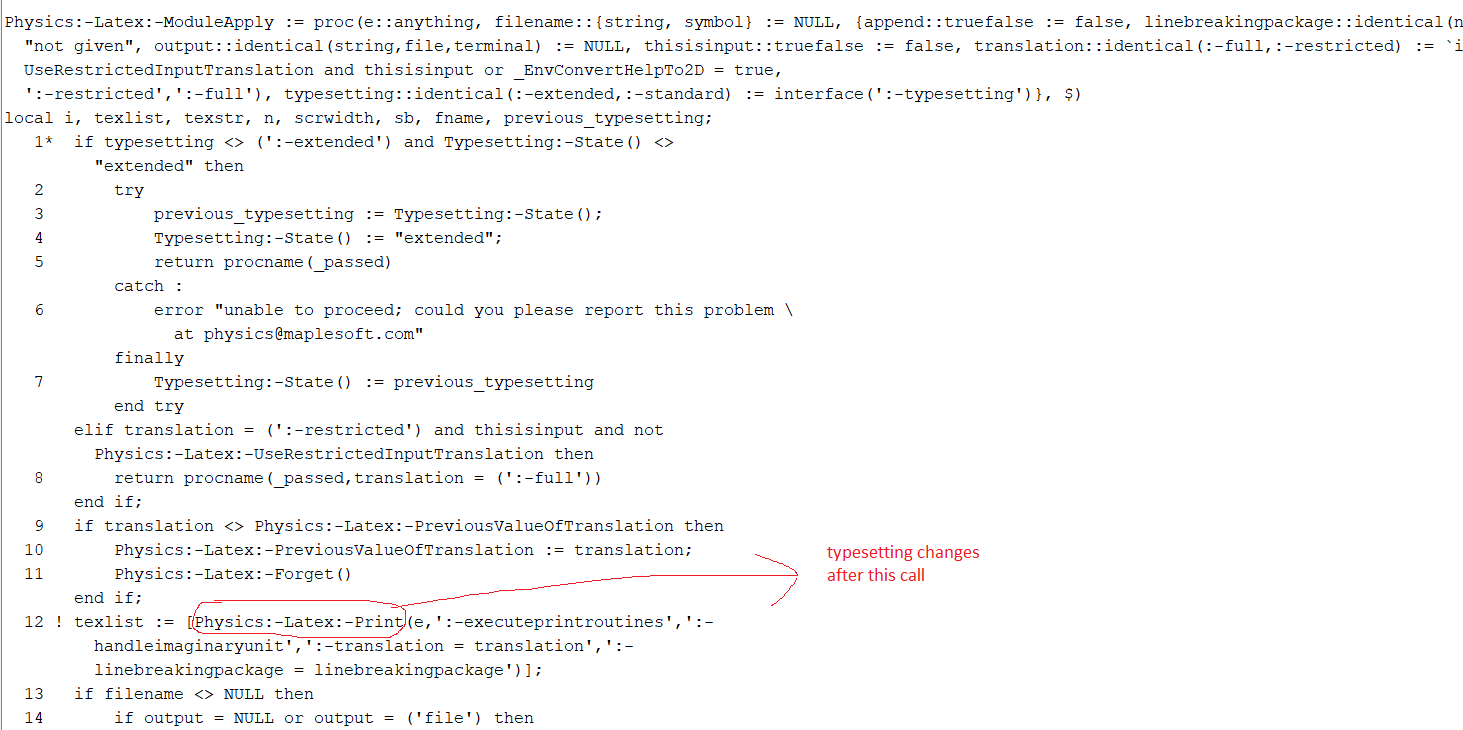# Question:new issue using Physics:-Latex() . Changes typesetting automatically.

## Question:new issue using Physics:-Latex() . Changes typesetting automatically.

Maple 2020

I spend all day on this, since some tests I have were failing when I changed to using Physics:-Latex() vs. latex(). I made no change at all in my own code other than to replace latex() by Physics:-Latex().

I am still not sure why that is, but it seems due to symbol clash somewhere.  First time I run my test, it passes, next time if fails. When I change back to latex(), the test passes each time.

This seems to be due to when calling Physics:-Latex() the very first time, it automatically changing diff(y(x),x) to y'(x). May be it is storing its own x in there. (this happens on special input and not for each input, that is why only some tests fail and not all of them).

So next time I call the test it failes. May be due to different x being used. I do not know.

All what I know now is that using latex() makes my dsolve test passes each time.

But here I will show the issue which I think if fixed, should fix the main problem.

Calling Physics:-Latex() causes it to automatically change diff(y(x),x) to y'(x)  even though I am not using Typeseeting at all.

```restart;
s:= y = u*x:  #special case input which causes the typesetting to kick in
ode:=diff(y(x),x)=1:
print(ode);
Physics:-Latex(s,output = string):
print(ode);

```Notice how the derivative changes on its own to using y'(x).  Now compare with latex()

```restart;
s:= y = u*x:
ode:=diff(y(x),x)=1:
print(ode);
latex(s,output = string):
print(ode);
```So something happens inside Physics:-:Latex which causes this.

For now, I will change back to latex() until I figure more what is going on so that my test do not fail. I like to use Physics:-:Latex as it made the latex look much better.

Maple 2020.1, Physics 778

 > restart;
 > interface(version)> Physics:-Version();> s:= y = u*x: ode:=diff(y(x),x)=1: print(ode); Physics:-Latex(s,output = string): #latex(s,output = string): print(ode);>